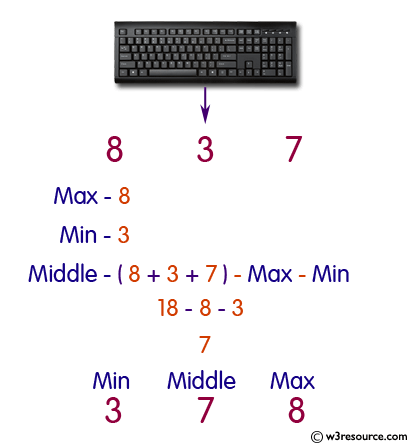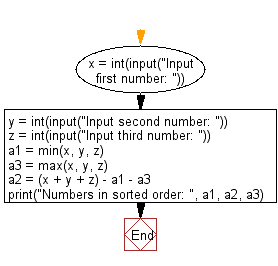﻿ Python: Sort three integers without using conditional statements and loops - w3resource# Python: Sort three integers without using conditional statements and loops

## Python Basic: Exercise-69 with Solution

Write a Python program to sort three integers without using conditional statements and loops.

Pictorial Presentation:Sample Solution:-

Python Code:

``````x = int(input("Input first number: "))
y = int(input("Input second number: "))
z = int(input("Input third number: "))

a1 = min(x, y, z)
a3 = max(x, y, z)
a2 = (x + y + z) - a1 - a3
print("Numbers in sorted order: ", a1, a2, a3)
```
```

Sample Output:

```Input first number: 2
Input second number: 4
Input third number: 5
Numbers in sorted order:  2 4 5
```

Flowchart:## Visualize Python code execution:

The following tool visualize what the computer is doing step-by-step as it executes the said program:

Python Code Editor:

Have another way to solve this solution? Contribute your code (and comments) through Disqus.

What is the difficulty level of this exercise?

Test your Python skills with w3resource's quiz

﻿

## Python: Tips of the Day

Try-catch-else construct:

```try:
foo()
except Exception:
print("Exception occured")
else:
print("Exception didnt occur")
finally:
print("Always gets here")
```